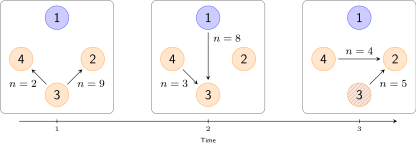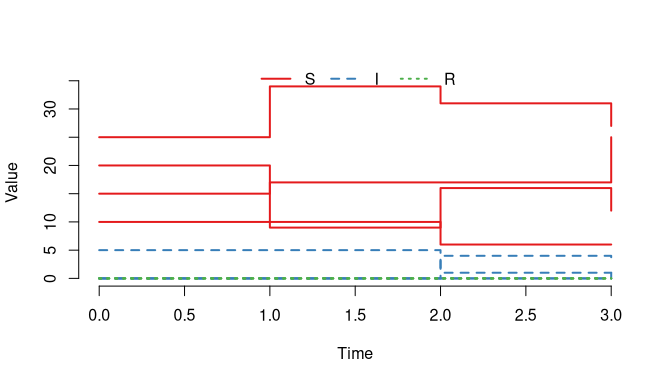# Scheduled events

NB: This vignette is work-in-progress and not yet complete.

## Overview

This vignette describes how births, deaths and movements can be incorporated into a model as scheduled events at predefined time-points. Events can, for example, be used to simulate disese spread among multiple subpopulations (e.g., farms) when individuals can move between the subpopulations and thus transfer infection, see Figure 1. In SimInf, we use node to denote a subpopulation.

$$~$$Figure 1. Illustration of movements between nodes. Each time step depicts movements during one time unit, for example, a day. The network has N=4 nodes where node 1 is infected and nodes 24 are non-infected. Arrows indicate movements of individuals from a source node to a destination node and labels denote the size of the shipment. Here, infection may spread from node 1 to node 3 at t=2 and then from node 3 to node 2 at t=3.

## A first example

Let us define the 6 movement events in Figure 1 to include them in an SIR model. Below is a data.frame, that contains the movements. Interpret it as follows:

1. In time step 1 we move 9 individuals from node 3 to node 2
2. In time step 1 we move 2 individuals from node 3 to node 4
3. In time step 2 we move 8 individuals from node 1 to node 3
4. In time step 2 we move 3 individuals from node 4 to node 3
5. In time step 3 we move 5 individuals from node 3 to node 2
6. In time step 3 we move 4 individuals from node 4 to node 2
events <- data.frame(
event      = rep("extTrans", 6),  ## Event "extTrans" is
##  a movement between nodes
time       = c(1, 1, 2, 2, 3, 3), ## The time that the event happens
node       = c(3, 3, 1, 4, 3, 4), ## In which node does the event occur
dest       = c(4, 2, 3, 3, 2, 2), ## Which node is the destination node
n          = c(9, 2, 8, 3, 5, 4), ## How many individuals are moved
proportion = c(0, 0, 0, 0, 0, 0), ## This is not used when n > 0
select     = c(4, 4, 4, 4, 4, 4), ## Use the 4th column in
## the model select matrix
shift      = c(0, 0, 0, 0, 0, 0)) ## Not used in this example

and have a look at the data.frame

events
##      event time node dest n proportion select shift
## 1 extTrans    1    3    4 9          0      4     0
## 2 extTrans    1    3    2 2          0      4     0
## 3 extTrans    2    1    3 8          0      4     0
## 4 extTrans    2    4    3 3          0      4     0
## 5 extTrans    3    3    2 5          0      4     0
## 6 extTrans    3    4    2 4          0      4     0

Now, create an SIR model where we turn off the disease dynamics (beta=0, gamma=0) to focus on the scheduled events. Let us start with different number of individuals in each node.

library(SimInf)

model <- SIR(u0 = data.frame(S = c(10, 15, 20, 25),
I = c(5,  0,  0,  0),
R = c(0,  0,  0,  0)),
tspan = 0:3,
beta = 0,
gamma = 0,
events = events)

The compartments that an event operates on, is controlled by the select value specified for each event together with the model select matrix (E). Each row in E corresponds to one compartment in the model, and the non-zero entries in a column indicate which compartments to sample individuals from when processing an event. Which column to use in E for an event is determined by the event select value. In this example, we use the 4th column which means that all compartments can be sampled in each movement event (see below).

model@events@E
## 3 x 4 sparse Matrix of class "dgCMatrix"
##   1 2 3 4
## S 1 . . 1
## I . 1 . 1
## R . . 1 1

In another case you might be interested in only targeting the susceptibles, which means for this model that we select the first column. Now, let us run the model and generate data from it. For reproducibility, we first call the set.seed() function since there is random sampling involved when picking inviduals from the compartments.

set.seed(1)
result <- run(model)

And plot (Figure 2) the number of individuals in each node.

plot(result, range = FALSE)Figure 2. Number of susceptible, infected and recovered individuals in each node.

$$~$$

Or use the trajectory() function to more easily inspect the outcome in each node in detail.

trajectory(result)
##    node time  S I R
## 1     1    0 10 5 0
## 2     2    0 15 0 0
## 3     3    0 20 0 0
## 4     4    0 25 0 0
## 5     1    1 10 5 0
## 6     2    1 17 0 0
## 7     3    1  9 0 0
## 8     4    1 34 0 0
## 9     1    2  6 1 0
## 10    2    2 17 0 0
## 11    3    2 16 4 0
## 12    4    2 31 0 0
## 13    1    3  6 1 0
## 14    2    3 25 1 0
## 15    3    3 12 3 0
## 16    4    3 27 0 0